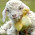# Java code to find frequency of each character in a String

Java program to find the frequency of each character in a String.

```import java.io.*;
class Frequency
{
static String n;
static int l;
public static void main(String args[]) throws IOException
{
BufferedReader br = new BufferedReader(new InputStreamReader(System.in));
System.out.print("Enter a String : ");
n = br.readLine();
l = n.length();
freq();
}
public static void freq()
{
int s=0,f=-1;
for(int i=0;i<l;i++)
{
// Find frequecy
for(int j=0;j<l;j++)
{
if(n.charAt(i)==n.charAt(j))
s++;
}

// Check if the letter has occured before
for(int k=0;k<i;k++)
{
if(n.charAt(i)==n.charAt(k))
f = 1;
}

// Print the letter's frequency
if(f==-1)
System.out.println(n.charAt(i) +" = " +s);
s=0;
f=-1;
}
}
}
```

ALGORITHM:-

1. Start

2. Accept a sentence from the user.

3. Extract a character.

4. Count the no. of times it occurs in the sentence.

5. Print its frequency.

6. Repeat Steps 3 to 6 till all the frequencies are printed.

7. End

OUTPUT:-

Enter a String : reverberate
r  = 3
e  = 4
v  = 1
b  = 1
a  = 1
t  = 1

Related Programs:-

Generate Fibonacci series using recursion

Find the HCF & LCM of two numbers using recursion

Find the sum of the following series using recursion : 12 + 22 + 32 +.......+ n2

Find the sum of Natural numbers up to a given number using Recursion

Calculate the Power of a number using recursion

#### 3 comments:

1.nice logic thanks a lot for help.

2.This is awesome!

3.java 2d graphic code examples
Drawing Shapes in Java